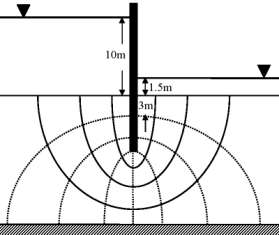# Civil Engineering: Hydrology and Water Resource Engineering MCQs

##### 1. Question

The annual precipitation data of a city is normally distributed with mean and standard deviation as1000 mm and 200 mm, respectively. The probability that the annual precipitation will be more than 1200 mm is

< 50%
50%
75%
100%

`Answer`
##### 2. Question

The ratio of actual evapo-transpiration to potential evapo-transpiration is in the range

0.0 to 0.4
0.6 to 0.9
0.0 to 1.0
1.0 to 2.0

`Answer`
##### 3. Question

The flow net around a sheet pile wall is shown in the sketch. The properties of the soil are: permeability coefficient = 0.09 m/day (isotropic), specific gravity = 2.70 and void ratio = 0.85. The sheet pile wall and the bottom of the soil are impermeableThe seepage loss (in m3 per day per unit length of the wall) of water is

0.33
0.38
0.43
0.54

`Answer`
##### 4. Question

The flow net around a sheet pile wall is shown in the sketch. The properties of the soil are: permeability coefficient = 0.09 m/day (isotropic), specific gravity = 2.70 and void ratio = 0.85. The sheet pile wall and the bottom of the soil are impermeableThe factor of safety against the occurrence of piping failure is

3.55
2.93
2.60
0.39

`Answer`
##### 5. Question

The drainage area of a watershed is 50 km2. The index is 0.5 cm/hour and the base flow at the outlet is 10 m3/s. One hour unit hydrograph (unit depth = 1 cm) of the  watershed is triangular in shape with a timebase of 15 hours. The peak ordinate occurs at 5 hours.

The peak ordinate (in m3/s/cm) of the unit hydrograph is

10.00
18.52
37.03
185.20

`Answer`
##### 6. Question

The drainage area of a watershed is 50 km2. The  index is 0.5 cm/hour and the base flow at the outlet is 10 m3/s. One hour unit hydrograph (unit depth = 1 cm) of the watershed is triangular in shape with a time base of 15 hours. The peak ordinate occurs at 5 hours.

For a storm of depth of 5.5 cm and duration of 1 hour, the peak ordinate (in m3/s) of the hydrograph is

55.00
82.60
92.60
102.60

`Answer`